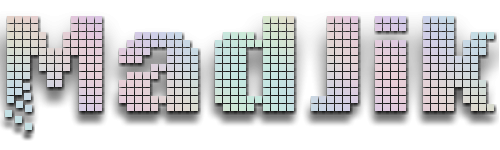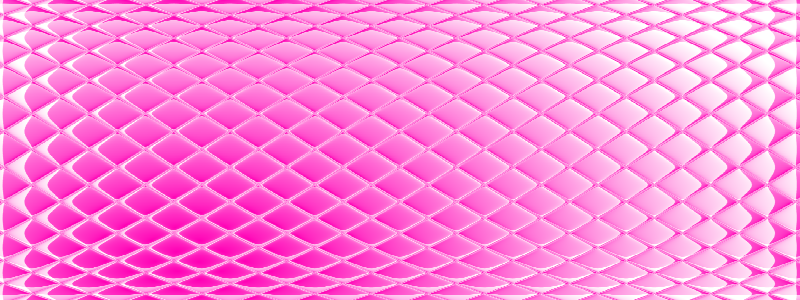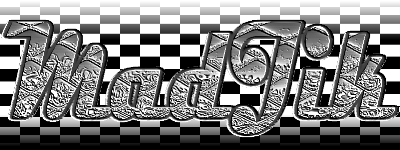# Cylinder effect : Looking for math help!

## Recommended Posts

I'm playing around with the codelab to find an alternative to the sphere plugin. I want to have a cylinder effect. Just like the sphere but only on X axle or Y axle.

For now this is where I am:

```void Render(Surface dst, Surface src, Rectangle rect)
{
float XDiv = dst.Width;
float YDiv = dst.Height;
float XCoef = XDiv / 2.0f;
float YCoef = YDiv / 2.0f;
float Pi = (float)Math.PI;
float XDecal = Pi / 2.0f;
float YDecal = Pi / 2.0f;
int mode = 2; // selection 0=X & Y, 1=X, 2 = Y

for(int y = rect.Top; y < rect.Bottom; y++)
{
for (int x = rect.Left; x < rect.Right; x++)
{
int srcX = (int)(XCoef + (Radius * XCoef * (Math.Tan(XDecal + (Pi * x / XDiv)))));
int srcY = (int)(YCoef + (Radius * YCoef * (Math.Tan(YDecal + (Pi * y / YDiv)))));
if(mode==0) dst[x, y] = src.GetBilinearSample(srcX,srcY);
if(mode==1) dst[x, y] = src.GetBilinearSample(srcX,y);
if(mode==2) dst[x, y] = src.GetBilinearSample(x,srcY);
}
}
}
```

Could someone check my formulas and help me to understand them?

This effect doesn't refill the whole surface...

Best way to test it is to create a gradient and apply a tile reflection effect. Then copy/paste the code to the codelab...

Once this done, I'll create the GUI and DLL for the plugin!

EDIT: Code last version:

```void Render(Surface dst, Surface src, Rectangle rect)
{
float ImgTLx = dst.Width;
float ImgTLy = dst.Height;
float ImgHLx = ImgTLx / 2.0f;
float ImgHLy = ImgTLy / 2.0f;
float Pi = (float)Math.PI;
int mode = 2;

for(int y = rect.Top; y < rect.Bottom; y++)
{
for (int x = rect.Left; x < rect.Right; x++)
{
float wX = (float)Radius *((Pi * x / ImgTLx) - (Pi / 2));
float wY = (float)Radius *((Pi * y / ImgTLy) - (Pi / 2));
int srcX = (int)(ImgHLx + (ImgHLx * (Math.Tan(wX))));
int srcY = (int)(ImgHLy + (ImgHLy * (Math.Tan(wY))));
if(mode==0) dst[x, y] = src.GetBilinearSample(srcX,srcY);
if(mode==1) dst[x, y] = src.GetBilinearSample(srcX,y);
if(mode==2) dst[x, y] = src.GetBilinearSample(x,srcY);
}
}
}
```My DeviantArt | My Pictorium | My Plugins | Donate via Paypal

##### Share on other sites

On a simple image (gradient + tile reflection), effect with last cde version:My DeviantArt | My Pictorium | My Plugins | Donate via Paypal

##### Share on other sites

Kind of use for sigs...orMy DeviantArt | My Pictorium | My Plugins | Donate via Paypal

## Join the conversation

You can post now and register later. If you have an account, sign in now to post with your account.×   Pasted as rich text.   Paste as plain text instead

Only 75 emoji are allowed.

×   Your previous content has been restored.   Clear editor

×   You cannot paste images directly. Upload or insert images from URL.

×
×